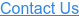# Swap Invoice Explanation

Swap invoices frequently cause confusion as they are billed separately from the loan.

#### Interest Rate Assumptions

LIBOR                         2.00%

Floating Rate Today  4.00%

Swap Rate                   2.50%

Fixed Rate                  4.50%

#### Swap Invoices Explained – CPA’s

##### Borrower Pays

Borrower pays on loan  4.00%

Borrower pays on swap 4.50%

Total                                  8.50%

Total                                         4.00%

The borrower’s effective fixed rate is therefore 8.50% – 4.00% = 4.50%.  This matches the swap rate quoted above.

Now, let’s assume LIBOR is at 6.00%.

##### Borrower Pays

Borrower pays on loan  8.00%

Borrower pays on swap 4.50%

Total                                12.50%

Total                                        8.00%

The borrower’s effective fixed rate is therefore 12.50% – 8.00% = 4.50%.  This matches the swap rate quoted above.

#### Swap Invoices Explained – Borrowers

##### Loan Payment

Borrower pays2.00% + 2.00% = 4.00%

##### Swap Payment

Borrower pays        2.50% + 2.00% = 4.50%

Borrower receives 2.00% + 2.00% = 4.00%

Borrower Net Pays                                0.50%

Add the two invoices together to determine the effective interest rate.

The effective rate is therefore 4.00% + 0.50% = 4.50%.  This matches the swap rate above.

Now, let’s assume LIBOR is at 6.00%.

##### Loan Payment

Borrower pays             6.00% + 2.00% = 8.00%

##### Swap Payment

Borrower pays        2.50% + 2.00% = 4.50%

Borrower receives 6.00% + 2.00% = 8.00%

The effective rate is 8.00% – 3.50% = 4.50%

#### Sample Payment Invoices with Principal – Mechanics

Assume a \$25mm loan with monthly principal of \$33,000 in a month with 30 days.

#### Interest Rate Assumptions

Floating Rate   4.00%

Floating interest payment will be \$25,000,000 * 4.00% * 30/360 = \$83,333.33.

Swap rate locked was 4.50%

Fixed interest payment will be \$25,000,000 * 4.50% * 30/360 = \$93,750.

Principal of \$33,000 per the Note.

With a traditional fixed rate loan of 4.50%, the monthly P&I payment should be \$126,671.33 as follows:

Principal  \$ 32,921.33

Interest    \$ 93,750.00

Total         \$126,671.33

How the actual mechanics will work:

##### Loan Invoice

Principal              \$ 32,921.33

Interest                \$ 83,333.33

Borrower Pays   \$116,254.66

##### Swap Invoice

Borrower pays          \$93,750.00